# Addressing Modes & CPU Internals

## 1  Introduction

• This week we get to the heart of the matter: exactly how does a CPU work.
• However, before that, we need to look at the possible addressing modes that designers have created for the operands of machine instructions.
• Remember, a CPU is designed so that a programmer can write programs on it, and these days most programs are written in high-level languages and translated down into machine code.
• Many of the addressing modes covered below make the job of a programmer easier, or make the task of translating high-level abstractions such as arrays, pointers, structures and objects easier.
• But there's a downside: more complex addressing modes require more memory accesses, thus making the instruction's duration longer, and also making the internal design of the CPU more complex.
• The RISC philosophy is to eschew all but the simplest of the addressing modes and write compilers to use these simple addressing modes efficiently.
• The CISC philosophy is to provide as many addressing modes as possible to make programming easier.

• These modes are used to modify the value in the Program Counter.

### 2.1  Absolute Jump

PC <- literal value in instruction
• i.e. the next instruction will be the one at the address defined by the literal value.
• This is used to jump the PC to the first instruction in a function, for example.
• The literal value is treated as unsigned, as there are no negative memory addresses.
• The size of the literal value sets the range of possible addresses.

### 2.2  Relative Jump (Branch)

PC <- old PC + literal value in instruction
• i.e. a branch.
• The literal value is treated as a signed value, so that the PC can jump backwards as well as forwards.
• Often, the branch instruction includes a comparison or condition, and the branch is only taken if the comparison is true, e.g.
```   beq \$t1, \$t2, 100      # PC = PC + 100 if \$t1 == \$t2

```
• This is used to skip around sections of code in IF .. THEN .. ELSE, or to branch backwards in short loops.
• In general, most branch distances are reasonably small, say only a few megabytes, so the value can be encoded in less bits than a full address. If necessary, chained relative jumps can be used to go further distances.

### 2.3  Register Indirect Jump

PC <- Rn
• i.e. the PC becomes the value in another register.
• The MIPS ISA uses this, for example, to return from a function call, by loading the PC with the return address \$ra register.

• In the following descriptions I will use a hypothetical 3-operand ADD instruction:
and use different addressing modes for Src2.
• Note that I am ignoring the Src1 and Dest operands. These, too, could use any of the following addressing modes.

• The operand's value is encoded in the instruction as a literal value.
• It may be treated as signed or unsigned depending on the instruction.
• MIPS example: addi \$t1, \$t2, 100
• Literal values are known on some architectures as immediate values.

### 3.2  Register Mode

• A register number is specified in the instruction; the operand's value comes from the register.
• MIPS example: add \$t1, \$t2, \$t3

### 3.3  Absolute or Direct Mode

• Note that most RISC ISAs only provide memory addressing for load & store instructions.
• CISC ISAs usually allow memory addressing for many instructions.
• MIPS example: lw \$t1, 5000

### 3.4  Register Indirect Mode

• The instruction encodes a register number.
• The value in the register is treated as an address; the operand's value is loaded from that address.
• The register is effectively a pointer: instead of holding a value, the register points at the location in memory where that value exists.
• MIPS example: lw \$t1, (\$t2)
• The idea of a pointer is used in many languages to create dynamic data structures, e.g. linked lists.
• In Java, a linked list of ints can be created with:
```    public class IntList
{
private int     x;
private IntList next;
}

```
although the next variable is strictly a reference, not a pointer: Java stops you from seeing the address.
• In C, we can do:
```    struct IntList
{
int             x;
struct IntList *next;
}

```
and the next variable is a pointer which holds an address and which is directly visible to the programmer.

### 3.5  Indexed Absolute Mode

• The instruction encodes a literal value, the base, and a register number.
• The register is known as an index register.
• MIPS example: lw \$t1, 300(\$t2)
• This allows the low-level implementation of arrays.
• Assume that an array of 25 characters, name[], is stored starting at memory address 50 onwards.
• The character name is stored at location 50, name at 51, name at 52 etc.
• A program can loop with register Rn going from 0 up to 24.
• The operand    50(Rn)    would access each of the characters in the array from position 0 up to 24.
• Note that, for arrays of different type sizes, e.g. 32-bit ints, the index register has to be incremented by the byte size of the elements (i.e. 4) each time through the loop.
• This addressing mode is useful when the array is fixed in memory: for example, it is a global array defined at compile time.
• The rest of the addressing modes below are seen mainly on CISC architectures.

### 3.6  Base plus Offset Mode

• This time, the offset is the fixed literal, and the base of the data structure is obtained from a register.
• This addressing mode is useful to access specific fields of a structure or object.
• Consider a structure of different-sized fields, e.g. a C struct:
```    struct Student
{
int id;          # 32-bit value
short age;       # 16-bit value
char gender;     # 8-bit value
}

```
• The id field is at offset 0 in the struct, the age field is at offset 4, and the gender field is at offset 6.
• Imagine you have a Student struct variable s1, and you want to set the age in the struct to 23:
```    s1.age = 23;

```
• If register R5 (playing the role of s1) points at the base of the struct, then the following would do the work:
```    StoreHalfword R5(4), 23

```
as we know the age field is 4 bytes from the address that R5 points to.

### 3.7  Base plus Index Mode

• Instead of encoding the base as a fixed literal value, the base value comes from a register. The values of the two registers are added together to form the address of the value to access.
• The base register is effectively a pointer to some data structure like an array, and the index pointer is the index into that array.
• This is useful when the data structure's location is not defined at compile-time, but is created at run-time by the program:
• the structure might be a local variable, created when the function starts.
• the structure might be dynamically allocated, e.g. with C's malloc() or Java's new operator.
• Assuming that the base register points at the base of the structure, this addressing mode can access the fields or elements in the structure.

### 3.8  Base plus Index plus Offset Mode

• The instruction encodes two registers and a literal value which is the offset
• The values of all three are added together to form the address of the value to access.
• This addressing mode is useful when there is an array of structs/objects, and you want to access a specific field within one of the objects, e.g.
```    studentlist.age = 23;

```
• The base register would point at the base of the array, the index would hold 57 * sizeof(Student), and the offset would be the offset of the age field from the base of each struct/object.

### 3.9  Register Pre-increment/Pre-decrement Mode

ADD Dest, Src, +Register or -Register
• The value in the register is pre-incremented or pre-decremented before it is used.
• This mode often appears in combination with the other addressing modes above.
• Used in many 1960s and 1970s ISAs, its use on the PDP-11 CPU inspired the pre-increment & pre-decrement operators in C, which have been adopted by its decendants like Java, i.e. ++x;

### 3.10  Register Post-increment/Post-decrement Mode

ADD Dest, Src, Register+ or Register-
• The value in the register is incremented or decremented after it is used.
• This mode often appears in combination with the other addressing modes above.

### 3.11  Memory Indirect Mode

• The value at the given memory address is fetched, and this is used as a pointer to the actual memory location.
• If you ever get to write a substantial amount of C programming, you will see this in action as a pointer to a pointer.
• As shown above, there is a single indirection: fetch the value to get the address, fetch the value at that address.
• On some systems, e.g. the PDP-10 minicomputer, the word size (i.e. data bus size) was much bigger than the address bus size, so there were extra bits left over in each word which were not needed as an address.
• One specific bit in a word was treated as an indirect bit:
• if it was set, then the CPU would treat the value as another address, and go and fetch the value at this address.
• This implies that you could chain addresses: one address could point at another one, which could point at a further one etc.
• One prank was to set the indirect bit in a word in memory, and put the address of that word in the word itself.
• Accesses to this location would cause the CPU to go into an endless loop of indirections.
• On some implementations of the PDP-10 ISA, this would lock the CPU up hard, and it would need to be powered down to fix the problem.

## 4  Implementation Issues of Addressing Modes

• At this point you might be saying: well this is all fine and good, but why learn all these addressing modes?
• First reason: most of the world is Intel IA-32, and it is CISC, and it provides a good many of the above addressing modes.
• At some point in your programming career, you will probably have to get down and dirty with some assembly programming, so knowing about these various modes will be useful.
• But the main reason is they will affect the implementation of the CPU, which is what we are going to look at next.
• Consider the MIPS instruction   add \$t1, \$t2, \$t3.
• One memory fetch brings the instruction into the CPU. All three register numbers are encoded in the instruction.
• All three registers are in the CPU. The CPU selects \$t2 and \$t3 and sends their values to the ALU to be added.
• The result coming out of the CPU is stored in the \$t1 register. Done.
• Now consider the MIPS instruction    lw \$t1, 300(\$t2).
• One memory fetch brings the instruction into the CPU. Both register numbers and the literal value are encoded in the instruction.
• To get the actual value to load, we firstly have to calculate the effective address.
• The 300 is sent to the ALU as one operand, and the value in \$t2 is sent as the second.
• The ALU is commanded to perform an ADD operation.
• The result from the ALU is now the effective address, but where is it stored? It needs to be stored in a temporary "holding" register in the CPU. This is sometimes known as an (effective) address register.
• Using the effective address register, a second memory fetch brings the actual value into the CPU.
• It can now be stored into the \$t1 register.
• The more complicated an addressing mode is, the more work has to be done by the CPU to implement it.
• Also, a single machine code instruction (i.e. the interface seen by the programmer) actually has to be implemented internally as a sequence of operations to perform the whole instruction.

## 5  How the CPU Works

• We are now able to look at the innards of the CPU: how all the components work together, and how an incoming instruction is decoded and controls the CPU's components.
• To get to this point, we first needed to know about:
• the general architecture of a CPU: ALU, registers, instruction register, program counter.
• how the CPU is connected to memory: address, data and control busses.
• the idea of an instruction set architecture (ISA): what sort of instructions a CPU can do.
• how the ALU works, and how a set of control lines determines the operation to perform.
• the use of multiplexors to select one output from a set of inputs.
• addressing modes: the variety of register and memory access modes which a CPU designer may want to implement.
• All of the above will play a role in understanding how a CPU works internally.
• There is one thing left to look at before we can cover the workings of the CPU: sequential circuits.

## 6  Sequential Circuits

• The ALU is an example of a combinatorial circuit: its output is a combination of the boolean inputs, and some boolean algebra implemented by logic gates, to provide an output based solely on the current inputs.
• Theoretically, the ALU's output changes instantly when any one of its inputs changes. In reality, the output is delayed due to the reaction time of the gates and the length of the interconnects between them.
• Combinatorial circuits are stateless: their output depends only on their inputs, and not of the previous state of the circuit.
• Sequential circuits, on the other hand, do retain state. Their output is a function of their current or previous state, and their inputs.
• Consider a single register. It continually outputs its value, and also receives inputs so that it can be updated.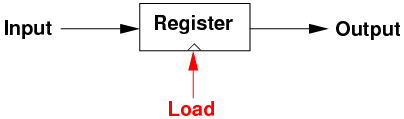• We don't want the register to change its value each & every time the input changes: all it would then do is echo the input straight to its output.
• Instead, we want to control when the input is loaded into the register, by asserting the load control signal.
• When the load signal is not asserted, the register retains its current state.
• Interestingly, registers and other sequential circuits can be built out of simpler sequential circuits known as flip-flops, which in turn can be built from simple logic gates.
• Combinatorial circuits have inputs which are are represented by their values: 0 or 1.
• This is true for the data inputs to sequential circuits, but the load signal is normally represented by a transition in value from 0 to 1.
• We say that a sequential circuit, such as a register, is edge-triggered: it updates its value when the load signal changes from 0 to 1.
• In some CPU designs, the control logic which tells the sequential circuits to load new values is asynchronous. This means that different sequential units will update at different times.
• For example, an ALU may output a new value, and also raise a control line's value from 0 to 1 at the same time. The output and the control line might be connected to a register, which will store the ALU value when the control line's value rises.
• But another register may be loaded from the data bus, which asserts (raises) a different control line at a different time.
• However, most CPU designs now are synchronous: all sequential units are updated at the same time.
• This synchronisation is controlled by a clock signal, usually a square wave generator which cycles between 0 and 1 values.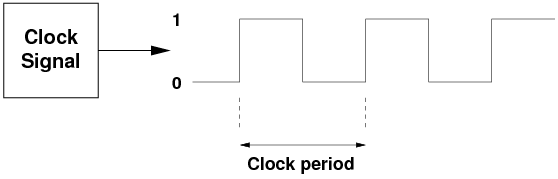• The clock's frequency and period are related by the equations Frequency*Period=1 or Period=[1/Frequency]
• If your CPU is running at 3GHz (i.e. 3 billion clock cycles per second, or 3*109clock cycles per second), then each clock period or clock cycle is 1/(3*109) seconds in duration, or about 0.33 nanoseconds (0.33*10-9) seconds long.
• On each rising or leading edge of the clock signal, sequential units in the CPU can be updated.
• However, we don't want them to be updated on every clock signal, only the ones when we have a new value to load.
• Therefore, the clock signal is usually ANDed with the update/load control line, for example: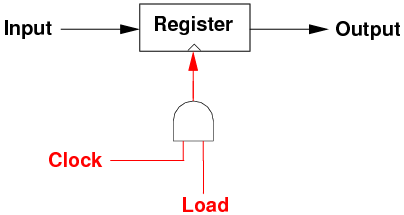• This ensures that all sequential units update at the same time, but only when needed.
• We put a small triangle at the bottom of each sequential unit, to indicate that it will receive a control signal to tell it when to update its contents based on the input.
• We will also leave out the clock signal and the AND gate, and just show the control line: they will be implicit.

### 6.1  Phases of Each Instruction

• You would think that we could simply wire the clock signal to each and every sequential unit in the PC, directly, and combine it with the control line which indicates updates. Unfortunately, this is not the case. Why not?
• The program counter (PC) holds the address of the next instruction. It's effectively a register, and is thus a sequential circuit.
• But each instruction has a number of phases, and we have to save the results of each phase. So the PC doesn't get updated on each phase of the instruction, just the last phase.
• Consider an instruction like MIPS   lw \$t1, 100(\$t0).
• On the first clock cycle, we must fetch and decode the instruction:
• Put the current PC value on the address bus, indicate a read on the read/write control line, receive and store the instruction into the instruction register (IR), which is a sequential circuit.
• On the second clock cycle, perform 100+ \$t0's value in the ALU:
• Get the 100 immediate value out of the IR, and \$t0's value, and apply as the two inputs to the ALU.
• On the third clock cycle, place the result from the ALU on the address bus, get the value at that location on the data bus, write the value on the data bus into \$t1.
• \$t1 is, of course, a sequential circuit.
• As well, increment PC, ready for the next instruction. The PC is a sequential circuit.
• Thus, we can't wire the clock signal directly in to all the sequential units in the CPU.
• Instead, we need a logic unit which receives clock cycles, and outputs control lines which are true (1) to indicate each phase of the instruction execution, and to reset itself after a certain number of cycles (e.g. 3).
• then we wire the appropriate phase lines to the different sequential units in the CPU.
• This unit has to keep state, so it is also a sequential circuit.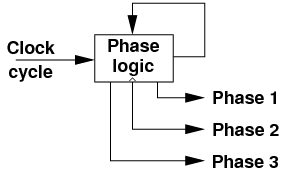• It is normally implemented as a shift register.
• We will see how these phase lines are used in the control logic below.

## 7  CPU Operation: Issues

• So far, we have seen a high-level overview of the CPU: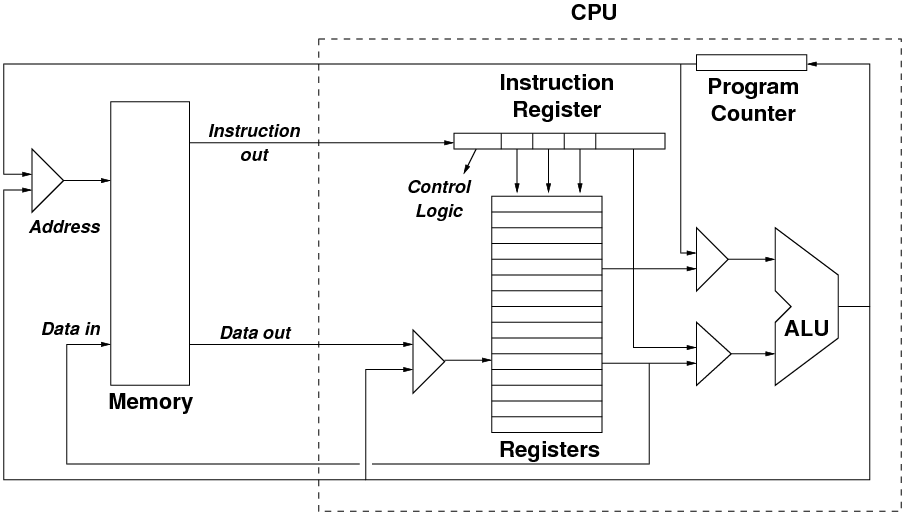• This diagram shows the datapaths only; it doesn't show the control logic.
• It also shows the CPU/memory interconnection as data flows, but in reality there are 3 busses which connect the CPU and memory: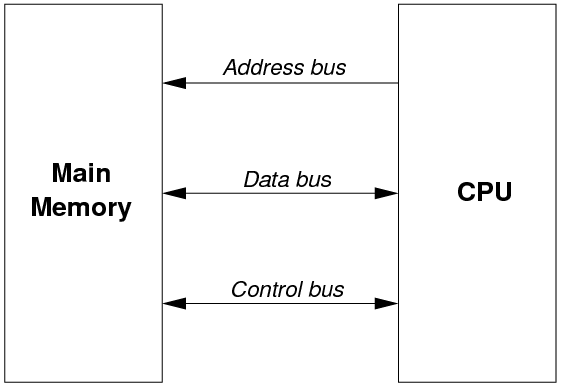• In particular:
• signals on the address bus flow only from the CPU to main memory
• signals on the data bus flow in both directions, to allow reading from and writing to memory
• some signals on the control bus flow from CPU to memory (e.g. the read/write signal), whereas others flow from memory to CPU (e.g. valid/invalid address).
• Let's look at some of the individual components in the CPU and see how they need to be controlled.

• The address bus sends the requested memory address out to main memory, so that location can be read or written.
• Along with this bus, there is a read/write line on the control bus which tells main memory what to do.
• Now, what sort of addresses can the CPU generate?
• the address of the instruction to fetch
• the address of a location to read or write, generated by an ALU operation (e.g. base + index)
• the address of a location to read or write, taken directly from a register
• We are going to need a multiplexor to control which address being generated in the CPU is the one that goes out on the address bus.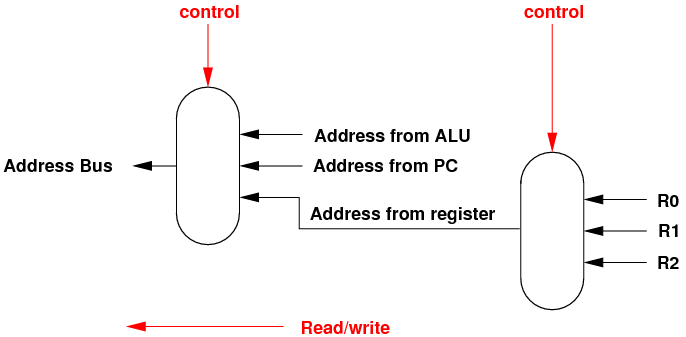• The instruction decoder will need to send control signals to the multiplexor to choose the address.
• Also note the right-hand multiplexor: if the address comes from a register, we need to choose which one.

### 7.2  The Data Bus

• The data bus is bi-directional, so we need a bi-directional multiplexor to determine the direction; it can be controlled by the read/write line.
• Where does write data come from?
• a register, e.g. load/store on RISC CPUs
• the result of the ALU operation, e.g. CISC instructions
• Where does read data go to?
• the instruction register (i.e. the fetch phase of an instruction)
• a register, e.g. load/store on RISC CPUs
• an input to the ALU, e.g. CISC instructions
• Fortunately, we can let the read data go in all directions, and let other multiplexors choose to accept/reject the read data.
• Again, the instruction decoder will need to send control signals to the data write multiplexor to choose the data to write.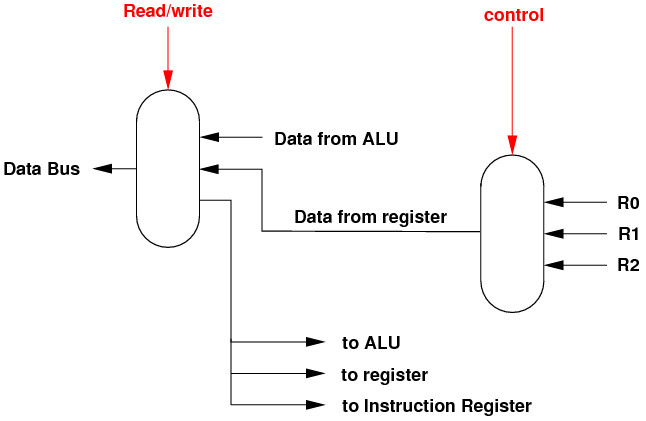### 7.3  The Program Counter

• The PC is a sequential circuit, and at the end of each instruction we need to update its value so as to fetch the next instruction.
• For normal flow of control, the PC needs to be incremented, so we need an incrementer unit.
• This could be as simple as a "add word size" operation, e.g. on RISC, or we need to keep track of the size of the current instruction, e.g. CISC instructions which are spread across multiple words.
• For jumps and branches, the address of the next instruction needs to be loaded into the PC.
• This can come from:
• a register, e.g. the jr instruction on MIPS to return out of a function.
• the ALU, which calculated a relative branch address using the old PC and some offset.
• the ALU, which calculated an absolute jump.
• an immediate value from the instruction register, i.e. a jump hard-coded in the instruction.
• Thus, we need a multiplexor and some control lines to determine how to update the PC.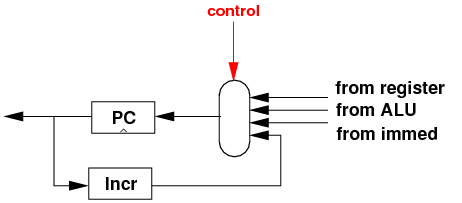### 7.4  The ALU

• The ALU is one of the most complicated units to control, mainly because it can get its two inputs from so many places:
• a specific register
• the data bus
• the PC, when we calculate branches
• an immediate value from the instruction register
• And as there are two inputs, we are going to need a multiplexor on each input to the ALU: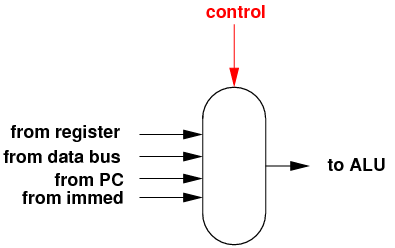• As well, the ALU needs control lines to determine its operation:• The output from the ALU will go to several other parts of the CPU, which will use multiplexors to choose/reject the ALU's output.
• Also note the status flags: individual bits which record the carry, overflow or zeroness of the result.
• These will be used by the branch logic to determine, for example, to modify the PC if the last result is zero (i.e branch if zero).

### 7.5  The Register File

• On some CPUs, certain registers are special-purpose: we are not going to look at that.
• Instead, we will consider all registers to be general-purpose and are numbered from zero upwards.
• The set of registers is known as a register file.
• The outputs from the registers can go off to many parts of the CPU, where multiplexors will select the values.
• We also need to control the inputs to the register file:
• if a write occurs or doesn't occur.
• to which register will the write go. We need a demultiplexor to do this.
• where will the data come from.
• For the latter, data can come from
• the ALU, e.g. MIPS   add \$t1, \$t2, \$t3
• the data bus, e.g. MIPS   lw \$t1, 1000
• an immediate value from the instruction register, e.g. load immediate value.
• the PC, to save the return address when doing a function call.
• Therefore, the control logic for the register file will look like this: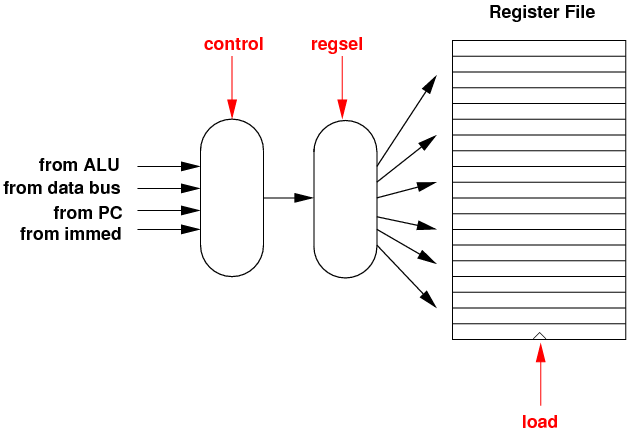### 7.6  Branch Logic

• We now come to the branch logic unit.
• On some CPUs, a branch instruction contains the desired comparison and the branch location, e.g. if R1 > R2, branch to PC+100.
• On other CPUs, the branch uses the status bits which are set by the last instruction, e.g. branch if zero to PC+100.
• Note that, for the first type, the ALU will do the comparison (usually by subtraction), and the branch logic has to determine the new address.
• In other words, the branch logic unit needs to have its own adder, to add the old PC and the offset to produce the new PC value.
• As inputs, the branch logic unit needs the zero status bit or the zero output from the ALU, an immediate value, and the old PC.
• The branch logic unit produces as output the new PC, and a bit to say if the comparison was true or false.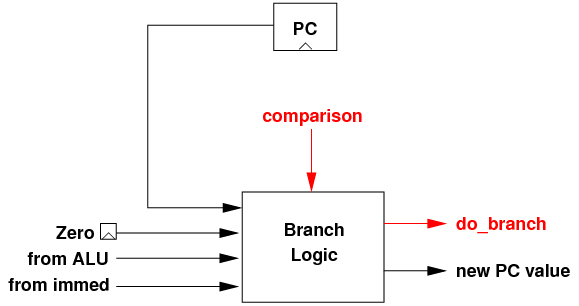• Note that this is just one possible unit. Other designs may take register values as inputs as well/instead of an immediate value etc.

### 7.7  The Instruction Register

• We now come to the instruction register, and preview the decode logic needed to interpret the instruction.
• Later on, we will look at two ways to do the full instruction decode and execution.
• Firstly, it's important to remember that, on most CPUs, there are different instruction formats, with different fields in each format.
• e.g. MIPS R-type, I-type and J-type formats.
• For each format, we need to have logic that extracts out the various fields.
• This can be as simple as selecting a subset of lines out from the IR.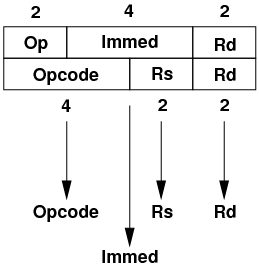• Some fields from the IR will be used as data (e.g. the immediate value), some as control lines.
• Then, we need to recognise the specific instruction format, and create several output lines which are true only for the specific format.
• On some CPU designs, fixed-position bits in every instruction can be used to determine the type of instruction.
• This can be done with a few logic gates.
• On other CPUs, a greater combination of bits in the instruction determine the type of instruction. More instruction bits need to go into the format decode unit, and more gates are needed inside, but this is not too difficult.

## 8  Hardwired Control Logic

• Given all of the above, you can see why the original overview diagram of the CPU internals only showed the datapaths and not the control logic. There would be too many red lines all over the place!
• One way to construct the control logic for a CPU is to hardwire the logic into the CPU using logic gates.
• The control logic not only has to use the decoded lines from the instruction, but it also has to be mindful that every instruction is a sequence of smaller activities which go on inside the CPU.
• The hardwiring needs to know when to assert the control lines to the units in the CPU, i.e. on which phase of the instruction.
• Revisiting the MIPS   lw \$t1, 100(\$t0)
• Phase 2: ALU input 1 <- 100 from IR, ALU input 2 <- register \$t0, ALU operation <- add
• Phase 3: address bus <- ALU output, read/write <- read, register select <- \$t2, register input <- data bus, register write <- true, PC <- incrementer, PC write <- true
• From the list, we can see that the address bus must select the PC as input on phase 1, the ALU as input on phase 3.
• Assuming that there are two control lines going into the address bus multiplexor, and they have this meaning:
 C1 C0 Meaning 0 0 ALU input 0 1 PC input 1 x Register input
then we can wire up the address bus multiplexor as follows: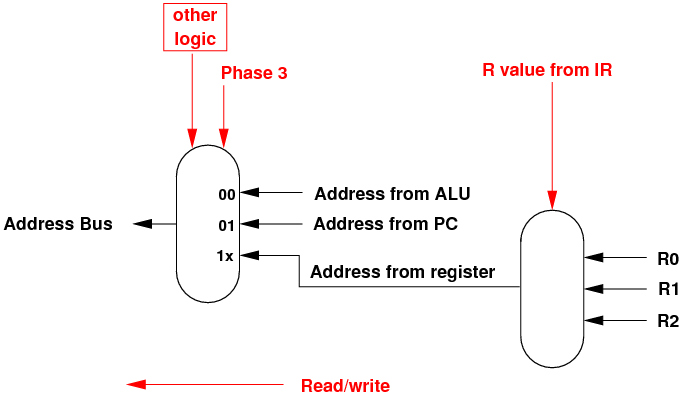• When Phase 3 is on, the PC is selected as input, i.e. C0=1. Otherwise, the ALU is the input (C0=0).
• The other control line, C1, is set using other logic gates only when a register is supplying the address on the bus.
• Each control input can thus be generated either by bits from the IR (e.g. register numbers), or by some combinatorial logic which ensures that the control line selects the right input, or writes the sequential unit, only on the appropriate phase of the instruction.
• The main advantage to use hardwiring to build the control logic for a CPU is that it only requires a few basic logic gates to represent the required combinations of when (which phase) and what (what input, allow write to sequential unit).
• One disadvantage is that it makes describing the control logic of the CPU nearly impossible, especially as a schematic diagram.
• In general, RISC CPUs are more likely to be hardwired whereas CISC CPUs tend to use microcode, described below.

## 9  Microcode Logic

• Before we press on, let's step back and consider: a CPU instruction involves a sequence of phases, and on each phase we need to either enable some control logic (send it a 1 bit), or route a specific value (e.g. a register number or a literal value) to the control logic.
• Instead of encoding all of this using dozens and dozens of logic gates which AND and OR to get the control lines right, why not store a table of control outputs in the CPU itself.
• Each row of the table would represent a single phase of a single instruction.
• Each column of the table would represent a specific control line, and the value which should be sent to it in this phase of the instruction.
• Implicitly, each real CPU instruction would be represented by a number of rows, and each row would be consulted in turn to perform the overall instruction.
• Going back to the MIPS   lw \$t1, 100(\$t0), the sequence of control lines verses the phases would be:
 Phase AddrBus R/W IR IRwr ALU1 ALU2 ALUop Regsel RegInp Regwr PC PCwr 1 PC R dbus 1 xx xx xx xx xx 0 xx 0 2 xx R xx 0 immed \$t0 ADD xx xx 0 xx 0 3 ALU R xx 0 xx xx xx \$t1 dbus 1 Incr 1
• This concept of storing a table of control line values inside the CPU is known as microcoding, or microprogramming.
• Each row is called a microinstruction. The whole table of microinstructions is known as the CPU's microprogram.
• As shown above, each microinstruction is followed by the next microinstruction. But we can increase the flexibility as follows:
• Give each microinstruction a number from 0, 1, 2 etc.
• Also add three more columns: a specific data or control value inside the CPU, and two "next microinstruction" numbers.
• If, after completing the microinstruction, the specified data value is true, then go to the first microinstruction number.
• Else, go to the second microinstruction number.
• This allows each microinstruction to choose the next instruction to perform, even to loop backwards!
• Some microprogram implementations allow a jump to a specific next instruction based on a set of input values, e.g. what particular opcode number is in the instruction register.
• This is the hardware equivalent of a high-level SWITCH statement: case value -> jump here.
• Most CPUs use microcoding. There is a big disadvantage:
• storing the table in the CPU needs many more logic gates than the equivalent hardwired control logic.
• But there are many advantages:
• the microinstructions are somewhat readable by humans, so the control logic can be understood.
• the CPU and its microcode can be simulated, so that any errors or bugs in the microcode can be found.
• the instruction set in the CPU is determined by the available sub-units (ALU, registers etc.) and by the values in the table. If a designer wants to change or extend the instruction set, they merely have to change the values in the table.
• some logic errors in a CPU design can be fixed by rewriting the microinstructions. CPUs like the Pentium family have the ability to rewrite the microprogram table after manufacture.
• by rewriting the microcode, frequently-used CPU instructions can be speeded up, maybe at the expense of other instructions.

### 9.1  Implementing the Microcode Logic

• In a sense, we are implementing a tiny CPU inside to control the real CPU.
• Here is an example of how it is implemented.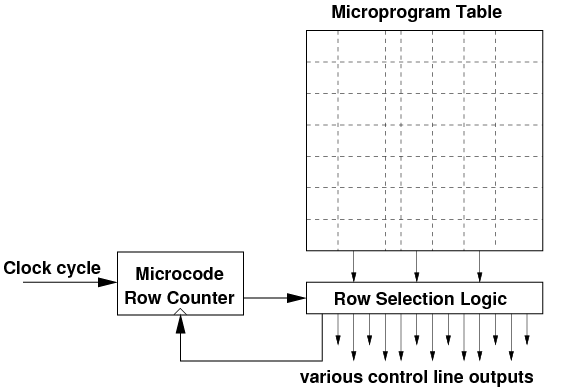• At each clock cycle, the microcode row counter holds the number of the next microinstruction to run (just like the PC).
• The row selection logic reads the table, extracts the row, and enables/disables each of the control output lines.
• If the microcode provides IF .. ELSE or SWITCH-style operations, then these are also performed.
• A new microcode row number is also output, and this is stored back into the row counter in preparation for the next clock cycle.
• All of the above probably seems abstract and esoteric. In the tutorial, we will look at an example of a hardwired CPU and also a graphical simulation of a microcoded CPU.

File translated from TEX by TTH, version 3.85.
On 12 Jan 2012, 15:50.